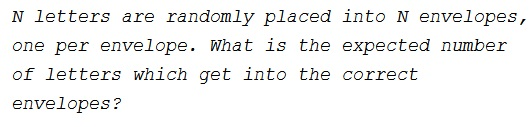Randomly Placed Letters in Envelopes

ProblemSolution 1

Each letter has the probability of $\displaystyle \frac{1}{N}$ to get into the right envelope. If $E_k$ is the event of the $k^{th}$ letter getting into the right envelope, i.e.,

$\displaystyle E_k=\begin{cases} 1,& \text{if the }k^{th}\text{ letter gets into the right envelope}\\ 0,& \text{otherwise}. \end{cases}$

The expectation of $E_k,$ is $\displaystyle E(E_k)=1\cdot\frac{1}{N}+0\cdot\frac{N-1}{N}=\frac{1}{N},$ for all $k.$

Although the events are not independent, the expectation being linear, we still have

$\displaystyle E\left(\sum_{k=1}^NE_k\right)=\sum_{k=1}^NE(E_k)=N\cdot\frac{1}{N}=1.$

Thus, for all $N$ the expected number of correct fits is $1.$ For $N=2,$ either both letters get into the right envelopes, or none does. Both events have the probability of $\displaystyle \frac{1}{2},$ with the expectation of

$\displaystyle E(E_1\bigcup E_2)=0\cdot\frac{1}{2}+2\cdot\frac{1}{2}=1.$

Acknowledgment

This is paraphrase of problem 104 from D. Newman's A Problem Seminar (Springer-Verlag, 1982).